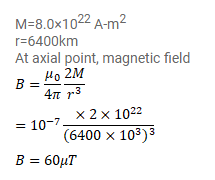# The magnetic moment of the assumed dipole at the earth's

Question:

The magnetic moment of the assumed dipole at the earth's center is $8.0 \times 10^{22} \mathrm{~A}-\mathrm{m}^{2}$. Calculate the magnetic field B at the geomagnetic poles of the earth. Radius of the earth is $6400 \mathrm{~km}$.

Solution: